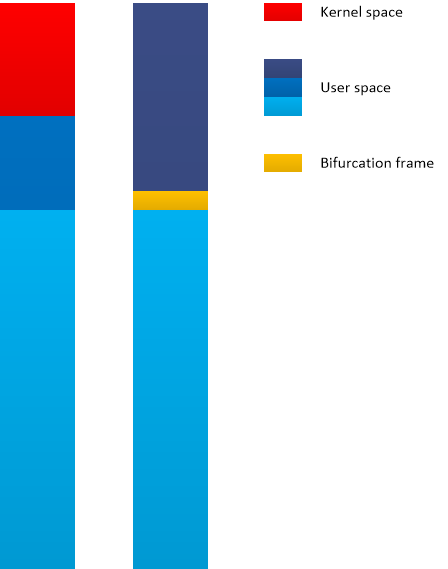## Crash Dump Analysis Patterns (Part 237)

Variable Subtrace analysis pattern was introduced for inter-correlational analysis of CPU spikes across memory snapshots with just one thread involved. In contrast, we found Constant Subtrace pattern useful in Wait Chain analysis involving several threads in just one memory snapshot (intra-correlational analysis). Here a constant subtrace groups stack traces from Stack Trace Collection with a bifurcation stack trace frame (similar to Bifurcation Point trace analysis pattern) providing some wait chain relationship hint. Such subtraces traces may be initially found by the preceding wait chain analysis or by technology-specific subtraces such as ALPC/RPC server thread frames (as seen in an example stack from COM interface invocation). Here is a minimal stack trace diagram (similar to minimal trace graphs introduced in Accelerated Windows Software Trace Analysis training) illustrating the pattern (it also shows Spiking Thread pattern in user space as seen from a complete memory dump):- Dmitry Vostokov @ DumpAnalysis.org + TraceAnalysis.org -